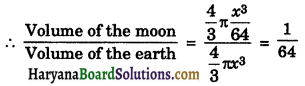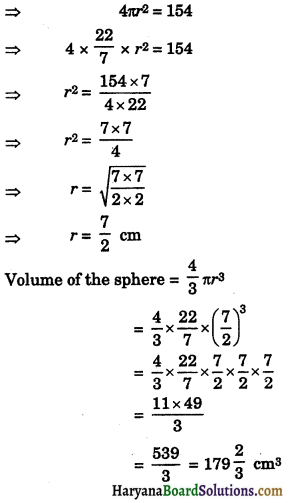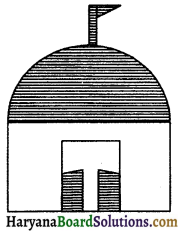# HBSE 9th Class Maths Solutions Chapter 13 Surface Areas and Volumes Ex 13.8

Haryana State Board HBSE 9th Class Maths Solutions Chapter 13 Surface Areas and Volumes Ex 13.8 Textbook Exercise Questions and Answers.

## Haryana Board 9th Class Maths Solutions Chapter 13 Surface Areas and Volumes Exercise 13.8

Assume π = $$\frac{22}{7}$$, unless stated otherwise.

Question 1.
Find the volume of a sphere whose radius is :
(i) 7 cm
(ii) 0.63 m.
Solution:
(i) We have,
Radius of sphere (r) = 7 cm
∴ Volume of sphere = $$\frac{4}{3}$$πr3
= $$\frac{4}{3} \times \frac{22}{7} \times(7)^3$$
= $$\frac{88 \times 49}{3}$$
= $$\frac{4312}{3}$$
= 4312$$\frac{1}{3}$$ cm3.
Hence,volume of the spher = 4312$$\frac{1}{3}$$ cm3.

(ii) We have,
Radius of the sphere (r) = 0.63 m
∴ Volume of the sphere = $$\frac{4}{3}$$πr3
= $$\frac{4}{3} \times \frac{22}{7} \times(0.63)^3$$
= 88 × 0.03 × 0.3969
= 1.0478 m3 = 1.05 m3
Hence,volume of the sphere = 1.05 m3.

Question 2.
Find the amount of water displaced by a solid spherical ball of diameter :
(i) 28 cm
(ii) 0.21 m.
Solution:
(i) We have,
Diameter of spherical ball = 28 cm
∴ Radius of the spherical ball (r) = $$\frac{28}{2}$$
= 14 cm
Amount of water displaced by spherical ball = Volume of spherical ball
= $$\frac{4}{3} \pi r^3=\frac{4}{3} \times \frac{22}{7} \times(14)^3$$
= $$\frac{4}{3} \times \frac{22}{7} \times 14 \times 14 \times 14$$
= $$\frac{88 \times 2 \times 196}{3}$$
= $$\frac{34496}{3}$$
= 11498$$\frac{2}{3}$$ cm3
Amount of water displaced by spherical ball
=11498$$\frac{2}{3}$$ cm3

(ii) We have,
Diameter of spherical ball = 0.21 m
∴ Radius of spherical ball (r) = $$\frac{0.21}{2}$$
= 0.105 m
Amount of water displaced by spherical ball = Volume of spherical ball
= $$\frac{4}{3}$$πr3
= $$\frac{4}{3} \times \frac{22}{7} \times(0.105)^3$$
= $$\frac{4}{3} \times \frac{22}{7}$$ × 0.105 × 0.105 × 0.105
= 88 × 0·005 × 0.011025
= 0.004851 m3
Hence, amount of water displaced by spherical ball = 0.004851 m3.

Question 3.
The diameter of a metallic ball is 4.2 cm. What is the mass of the ball, if the density of the metal is 8.9 g per cm3?
Solution:
We have,
Diameter of ball = 4.2 cm
∴ Radius of the ball (r) = $$\frac{4.2}{2}$$ = 2.1 cm
Since, ball is in the shape of sphere, therefore we need to calculate the volume of sphere for its mass.
∴ Volume of spherical ball = $$\frac{4}{3}$$πr3
= $$\frac{4}{3} \times \frac{22}{7} \times(2.1)^3$$
= $$\frac{88}{21}$$ × 2.1 × 2.1 × 2.1
= 8.8 × 4.41 = 38.808 cm3
mass of the ball = volume × density
= 38.808 × 8.9
= 345.39 grams (approx.)
Hence,mass of the ball = 345.39 grams (approx.)

Question 4.
The diameter of the moon is approximately one-fourth of the diameter of the earth. What fraction of the volume of the earth is the volume of the moon?
Solution:
Let the diameter of the earth be 2x.
∴ Radius of the earth (R) = $$\frac{2 x}{2}$$ = x
According to question,
Diameter of the moon = $$\frac{1}{4}$$ of diameter of the earth = $$\frac{1}{4}$$ × 2x = $$\frac{x}{2}$$
∴ Radius of the moon (r) = $$\frac{x}{4}$$
Volume of the earth = $$\frac{4}{3}$$πr3 = $$\frac{4}{3}$$πx3
Volume of the moon = $$\frac{4}{3}$$πr3 = $$\frac{4}{3} \pi\left(\frac{x}{4}\right)^3$$
= $$\frac{4}{3} \pi \frac{x^3}{64}$$⇒ Volume of the moon = $$\frac{1}{4}$$ × volume of the earth
Hence, $$\frac{1}{64}$$ fraction of volume of earth is volume of the moon.

Question 5.
How many litres of milk can a hemispherical bowl of diameter 10.5 cm hold.
Solution:
We have,
Diameter of hemispherical bowl = 10.5 cm
∴ Radius of hemispherical bowl (r) = $$\frac{10.5}{2}$$
= 5.25 cm
∴ Volume of the hemispherical bowl
= $$\frac{2}{3} \pi r^3$$
= $$\frac{2}{3} \times \frac{22}{7} \times(5.25)^3$$
= $$\frac{2}{3} \times \frac{22}{7}$$ × 5.25 × 5.25 × 5.25
= 44 × 0.25 × 27.5625
= 303.1875 cm3
= 0.3031875 litres
= 0.303 litres (approx.)
Hence, hemispherical bowl, can hold 0.303 litres (approx.) of milk.

Question 6.
A hemispherical tank is made up of an iron sheet 1 cm thick. If the inner radius is 1 m, then find the volume of the iron used to make the tank.
Solution:
We have,
Inner radius of the hemispherical tank (r) = 1 m
Thickness of the iron sheet = 1 cm
= 0.01 m
∴ Outer radius of the hemispherical tank
(R) = 1 + 0.01 = 1.01 m
Volume of the iron used to make the tank = External volume – Internal volume
= $$\frac{4}{3}$$πR3 – $$\frac{2}{3}$$πr3
= $$\frac{2}{3}$$π[R3 – r3]
= $$\frac{2}{3} \times \frac{22}{7}$$[(1.01)3 – (1)3]
= $$\frac{44}{21}$$(1.030301 – 1)
= $$\frac{44}{21}$$ × 0.030301
= 0·06348 m3 (approx.)
Hence, volume of the iron used to make the tank = 0·06348 m3 (approx.)

Question 7.
Find the volume of a sphere whose surface area is 154 cm2.
Solution:
Let the radius of sphere be r сm.
Surface area of the sphere = 154 cm2 (given)Hence,volume of the sphere = 179$$\frac{2}{3}$$ cm3.

Question 8.
A dome of a building is in the form of a hemisphere. From inside, it was whitewashed at the cost of Rs. 498.96. If the cost of the white-washing is Rs. 2.00 per square metre, find the :
(i) inside surface area of the dome,
(ii) volume of the air inside the dome.
Solution:Let inner radius of dome be r m.
We have, Rate of white washed = Rs. 2.00 per m2
Total cost of white washed = Rs. 498.96
(i) Inside curved surface area of the dome
= $$\frac{\text { Total cost }}{1 m^2 \cos t}=\frac{498.96}{2}$$

(ii) We have,
Inside C.S.A. of the dome = 249.48
2πr2 = 249.48
2 × $$\frac{22}{7}$$ × r2 = 249.48
r2 = $$\frac{249.48 \times 7}{22 \times 2}$$
r2 = 39.69
r = $$\sqrt{39.69}$$
r = 63 m.
Volume of air inside the dome = $$\frac{2}{3}$$πr3
= $$\frac{2}{3} \times \frac{22}{7} \times(6.3)^3$$
= $$\frac{2 \times 22}{21}$$ × 6.3 × 6.3 × 6.3
= 44 × 0.3 × 39.69
= 523.908 m3
= 523.9 m3 (approx.)
Hence, (i) Curved surface area of the dome = 249.48 m2
(ii) Volume of air inside the dome = 523.9 m3 (approx.)

Question 9.
Twenty seven solid iron spheres, each of radius r and surface area S are melted to form a sphere with surface area S’. Find the :
(i) radius r’ of the new sphere,
(ii) radio of S and S’.
Solution:
We have,
Twenty seven spheres, each of radius r and surface area S are melted to form a sphere with surface area S’.
(i) Then S = 4πr2 …..(i)
Volume of one sphere = $$\frac{2}{3}$$πr3
Volume of 27 spheres = 27 × $$\frac{2}{3}$$πr3
Volume of new sphere = Volume of 27 spheres
⇒ $$\frac{4}{3} \pi r^{\prime} 3=27 \times \frac{4}{3} \pi r^3$$
⇒ $$r^{\prime 3}=\frac{27 \times \frac{4}{3} \pi r^3}{\frac{4}{3} \pi}$$
⇒ r’3 = 27r3 = (3r)3
⇒ r’= 3r
(ii) Surface area of new sphere (S’) = 4πr’2
= 4π × (3r)2 = 4π × 9r2
= 36πr2
Now, $$\frac{S}{S^{\prime}}=\frac{4 \pi r^2}{36 \pi r^2}$$
⇒ $$\frac{S}{S^{\prime}}=\frac{1}{9}$$
⇒ S : S’ = 1 : 9
Hence (i)Radius of new sphere (r’) = 3r
(ii) S : S’ = 1 : 9.

Question 10.
A capsule of medicine is in the shape of a sphere of diameter 3.5 mm. How much medicine (in mm3) is needed to fill this capsule?
Solution:
We have,
Diameter of spherical capsule = 3.5 mm
∴ Radius of spherical capsule (r) = $$\frac{3.5}{2}$$
= 1.75 mm
Volume of the capsule = $$\frac{4}{3}$$πr3
= $$\frac{4}{3} \times \frac{22}{7} \times(1.75)^3$$
= $$\frac{4}{3} \times \frac{22}{7}$$ × 1.75 × 1.75 × 1.75
= $$\frac{88 \times 0.25 \times 3.0625}{3}$$
= $$\frac{67.375}{3}$$
= 22.46 mm3 (approx.)
Hence, volume of medicine in the capsule = 22.46 mm3 (approx.)